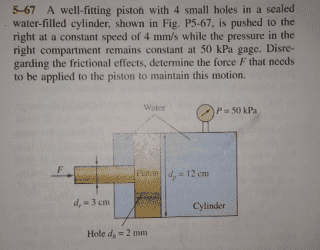# Fluid Mechanics-Bernoulli and Energy Equations

• Joseph95

## Homework StatementA well-fitting piston with 4 small holes in a sealed water-filled cylinder,shown in Fig is pushed to the right at a constant speed of 4 mm /s while the pressure in the right compartment remains constant at 50 kPa gage. Disregarding the frictional effects,determine the force F that needs to be applied to the piston to maintain this motion.

## Homework Equations

Bernoulli Equation: P1/ρ + V1^2/2 + gz1 = P2/ρ + V2^2/2 + gz2

Flow Rate : Q1 = Q2 V1.A1=V2.A2

## The Attempt at a Solution

I set two points.The first point (1) is back of the piston and the other point (2) front of the piston.So applied Bernoulli Equation:

P1/ρ + V1^2/2 +gz1 = P2/ρ + V2^2 / 2 + gz2 No elevation difference z1=z2

P1/ρ + V1^2/2 = P2/ρ + V2^2/2 V1=4 mm/s =0.004 m/s
V2= V1.A1/A2 = 0.004 x 0.0113 / 0.0000031 m/s
V2=3.64 m/s but there are 4 small holes so V2/4= 14.58/ 4 m/s=3.64 m/s

P1= P2 + ρV2^2/2 (V1 can be neglected)

P1= 50 kPa + 1000 (3.64)^2 /2 Pa = 56624.8 Pa

F/πDr^2/4=56624.8 Pa

F=40 N